# Random Forest Algorithm In Machine Learning

Random forests is a supervised learning algorithm in machine learning that can be used to solve both classifications and regression problems. It is popularly applied to data science competitions and practical, real-life situations and provides very intuitive and heuristic solutions. This article aims to give you a holistic and intuitive understanding of how this algorithm works.

## Key takeaways from this blog

• How does Random Forest & Bagging work?
• What is bootstrapping in Bagging & Random Forest?
• What are the hyperparameters involved with Random forest and their tuning for optimal performance?
• Feature Importance (Embedded Method).
• Implementation of Random Forests in python using Scikit-learn.

Random Forests leverages the power of Decision Trees, which is also the building block for it. If you have not checked our Decision Trees blog yet, we recommend looking for a concrete understanding.

## Random Forest

Decision trees work well on training data but poorly over the testing dataset. In other words, decision trees are prone to overfitting, especially when a tree is particularly deep. Hence, a single Decision Tree might not be the best fit for complex real-life problems. Then why not make multiple decision trees and then make a conclusive decision based on all DTs predictions? That’s where Random forest comes into the picture.

Random forest is a flexible, easy-to-use supervised machine learning algorithm that falls under the Ensemble learning approach. It strategically combines multiple decision trees (a.k.a. weak learners) to solve a particular computational problem. If we talk about the ensemble approach, the two most popular ensemble methods are Bagging and Boosting. To understand the Random Forest, we require the Bagging approach. So, let’s learn about bagging in detail.

## What is bagging?

Bagging, also called Bootstrap Aggregating, is a machine learning ensemble technique designed to improve the stability and accuracy of machine learning algorithms. It helps in eliminating the overfitting by reducing the variance of the output. To create a firm understanding of how bagging works, we should understand how bootstrapping works in bagging, which helps bagging techniques reduce overfitting.

### What is Bootstrapping in Bagging and Random Forest?

Bootstrapping is a statistical technique used for data resampling. It involves iteratively resampling a dataset with replacement. This statement is widespread and can be found in every definition of bootstrapping. The objective is to create multiple training datasets by collecting random samples from the original training set. Generally, we remove a sample from the subsequent trial once a sample gets selected in a random trial. But in bootstrapping, we do not do that. The same sample has an equal probability of getting selected for the subsequent trials, so we call it iteratively resampling a dataset with “replacement”.

In other words, we will create various datasets by selecting random samples from the original training set. A single observation might appear multiple times in the new bootstrapped dataset. The number of observations in the bootstrapped dataset would equal the original training set’s number of observations. These so formed bootstrapped training datasets are used in training the weak learners. The bootstrap technique helps in reducing the variance of the predictions, which vastly improves the overall predictive performance.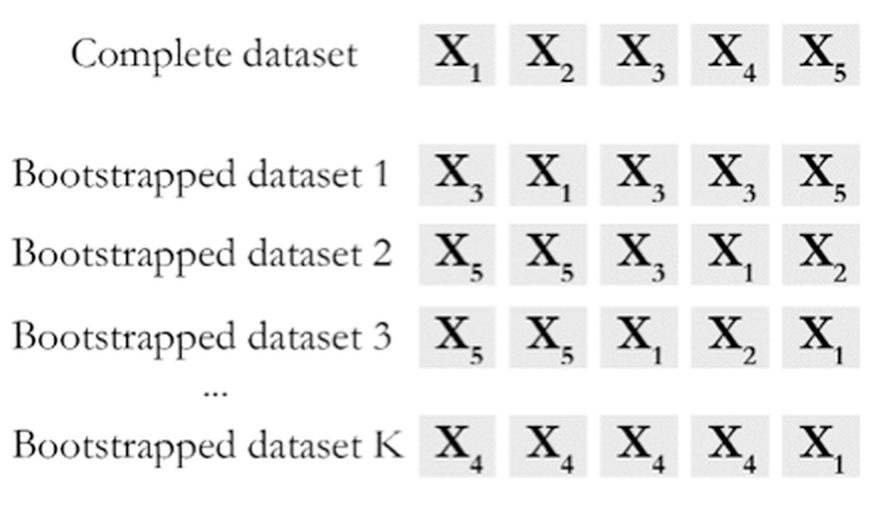Now, we have enough understanding of bootstrapping. Let’s continue with bagging. So, Bagging comprises of following three steps that are more or less common in Random Forest:

• Bootstrapping: The bootstrap method involves iteratively resampling a dataset with replacement. It helps create diverse samples that are usable for training weak learners.
• Parallel Training: Bootstrap samples are trained independently and parallel with each other using weak learners.
• Aggregation: In the case of classification, majority voting is performed to compute prediction. In regression, an average is calculated of all the outputs predicted by the individual weak learners.

The training algorithm for random forests applies the general bagging technique to tree learners with just one modification. Let's get back to the Random Forest.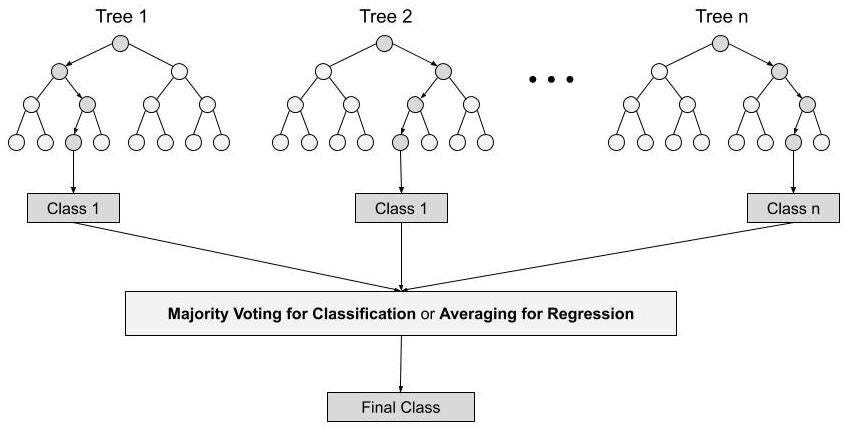### Continuing the discussion on Random Forest

Random forest builds multiple decision trees (weak learners) and merges their predictions to get a more accurate and stable prediction rather than relying on an individual decision tree.

The fundamental idea behind a random forest is to combine the predictions made by many decision trees into a single model. Individually, predictions made by decision trees may not be accurate, but when combined, the predictions will be closer to the actual value as it will give a scope of landing in the position of global optima for the cost function used for classification or regression problems.

Random Forest combines the simplicity of Decision Trees with flexibility resulting in vastly improved accuracy. But we must be thinking,

## What is the difference between Bagging and Random Forest?

In Random Forest, only a subset of features is selected at random out of total features, and the best split feature from the subset is used to split each node in a tree, whereas in bagging, all features are considered for splitting a node. Random Forest is a natural extension of Bagging. For example, one dataset has ten different features, but when we build DTs for the random forest, we will use only 3 out of 10.

## Let’s build Random Forest step by step

### Step 1.Create bootstrapped datasets

Multiple Bootstrapped datasets are created by drawing samples randomly with replacements from the original dataset. The same observations can appear more than once. The number of observations is the same in bootstrapped and the original dataset.

``````bootstrap = True
# When Bootstrapping is false, each tree will use the same dataset
# without randomness.
``````

### Step 2. Build a Decision tree on the Bootstrapped dataset

Build decision trees over the bootstrapped datasets formed in the previous step, but these decision trees only consider a random subset of variables for each split. Generally, this number is decided by the square root of the total number of features in the original dataset, and this can be tuned for optimal performance. If there are 36 independent features in the training dataset, Random Forest will randomly select six to build the decision trees. A variant of log(#features) is also popular in industries. These choices are popular because scientists experimented with several datasets and concluded the same.

``````max_features = sqrt(n_features)
``````

### Step 3. Aggregation

Finally, samples are fed through the trained Decision Trees for classification, where all the decision trees make classifications. Lastly, majority voting determines the new sample's final class. In the regression task, the prediction is calculated by averaging the predictions of the decision trees producing the final prediction.

The figure below illustrates how the Random Forest algorithm works.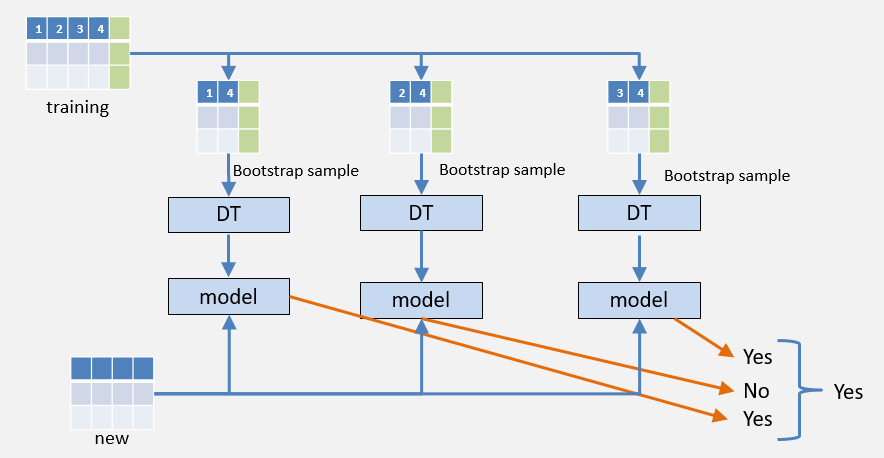Statistically, 1/3rd of original data never gets reflected in the bootstrapped dataset. This is known as the “Out-of-Bag Dataset”.

## How to test the accuracy of Random Forest?

Not all the data points from the original dataset are reflected in the Bootstrapped dataset. Such data points are collectively known as Out-of-Bag Dataset and can be used for testing the accuracy of the Random Forest. We can measure the accuracy of our Random Forest model by the proportion of Out-of-Bag samples that were correctly classified by it. On the contrary, the proportion of incorrectly classified samples is referred to as "Out-of-Bag Error."

Now, as we know most of the things about this algorithm, let’s quickly see one important support that it provides, i.e.,

### Feature Importance In Random Forest

Feature Importance is another reason for using Random Forest. In feature selection, embedded methods are known for their high performance. The embedded method requires an algorithm for the computation of feature importance. No doubt, Random Forest performs well in determining the relative feature importance, unlike the linear models.

It describes the dependency of the independent parameters over the target class by assigning relative importance.

There are many, but let’s list down some important ones,

• Reduction in overfitting - The bagging method significantly lowers the risk of overfitting.
• Minimal data cleaning efforts are required.
• Acceptable results even without tuning the hyper-parameters.
• It can be used for both regression and classification tasks.
• Out-of-Bag data can be used as a validation set for the model. So, no need to segregate the data for train and testing.
• Measures the relative importance of each feature on the prediction.

## Hyperparameters in Random Forest Algorithm

Let's get familiar with the Hyperparameters of Random Forest, which we will need for tuning the performance of the Random Forest algorithm.

• n_estimators: Number of trees in the Random Forest.
• max_features: The number of features to consider when looking for the best split.
• minisampleleaf: The minimum number of samples required to be at a leaf node.
• max_depth: The maximum depth of the tree. If none, then nodes are expanded until all leaves are pure.
• minsamplessplit: The minimum number of samples required to split an internal node.
• oob_score: OOB stands for Out-of-Bag. It is the score obtained by evaluating the model over the out of Bag samples. These samples were not used in the model building and can be used as a validation set.

Enough theory. Let's see Random Forest in action!

## Basic Implementation of Random Forest Algorithm

### Dataset Explanation

For the demonstration purpose, we will use the Pima Indian Diabetes Dataset. This is a supervised dataset used to classify the patients as diabetic or non-diabetic.

``````# Importing libraries
import graphviz
import pandas as pd
import numpy as np
import matplotlib.pyplot as plt
from sklearn import tree
from sklearn.model_selection import GridSearchCV
from sklearn.ensemble import RandomForestClassifier

``````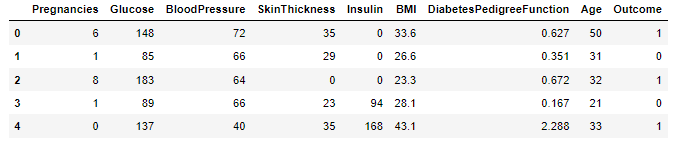### Fitting the Random Forest model

``````X = df.drop('Outcome',axis=1)
y = df['Outcome']

# Initialize Base-Line Random Forest Model
classifier = RandomForestClassifier(random_state=90, oob_score = True)
# Fitting data over the baseline model
classifier.fit(X, y)

# Evaluate the performance over the Out-of-Bag dataset
classifier.oob_score_

# 0.7734375
``````

We have achieved 77.34% accuracy without any hyper-tuning! Let's see how far we can improve this using hyper-parameter tuning.

### Hyperparameter Tuning for Random Forest

``````# Define the parameter Grid
params = {
'max_depth': [15, 20, 25],
'max_features': ['auto', 'sqrt'],
'min_samples_split': [10, 20, 25],
'min_samples_leaf': [5, 10],
'n_estimators': [10, 25, 30]
}

# Initialize the Grid Search with accuracy metrics
grid_search_result = GridSearchCV(estimator=classifier,
param_grid=params,
cv = 5,
scoring="accuracy")

# Fitting 5 Folds for each of 108 candidates, total 540 fits
# Fit the grid search to the data

grid_search.fit(X, y)

# Let's check the score
grid_search.best_score_

# 0.783868
# 1.35% improvement
``````

Accuracy has slightly improved!! The tuned model has 1.35% better accuracy than the baseline model.

``````# Let's check the parameters of our best model
best_model = grid_search.best_estimator_
print(best_model)

# RandomForestClassifier(max_depth=15, min_samples_leaf=10,
#      min_samples_split=25, n_estimators=25, random_state=90)
``````

### Now visualize the tree!!

``````# Visualize a single Decision Tree having depth = 4
dot_data = tree.export_graphviz(best_model.estimators_,
out_file=None,
feature_names=X.columns,
class_names="Outcome",
filled=True)

graph = graphviz.Source(dot_data, format="png")
graph
``````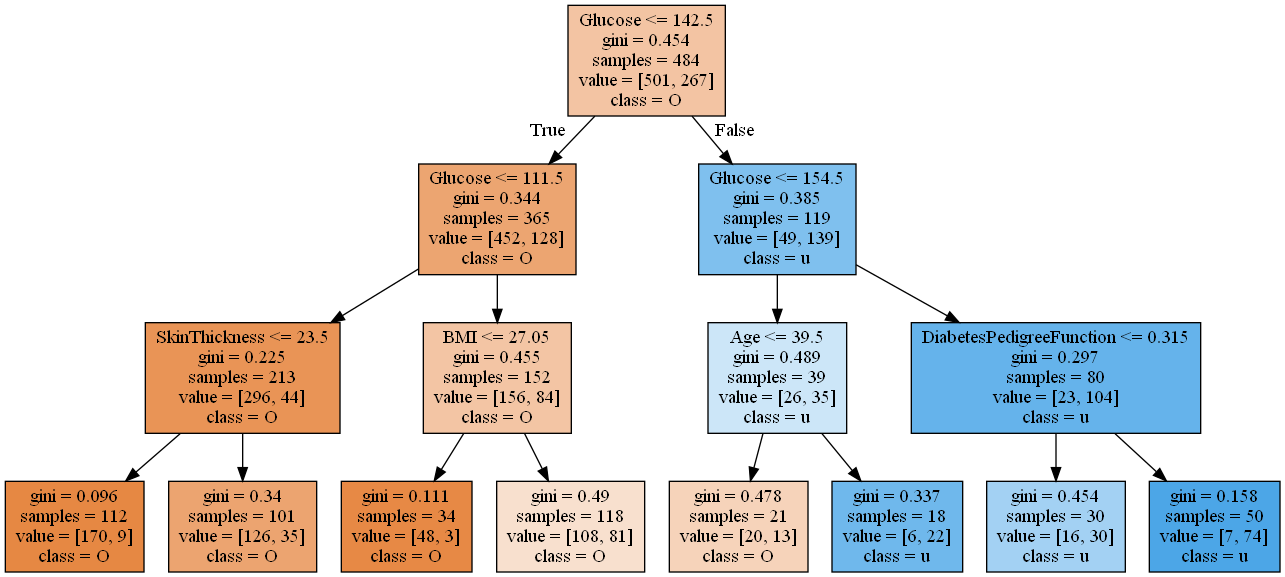### Relative Feature Importance as per the tuned model

``````# Plotting the Relative Importance as per tuned model
feature_importance = best_model.feature_importances_
feature_importance = 100.0 * (feature_importance / feature_importance.max())
sorted_idx = np.argsort(feature_importance)
sorted_idx = sorted_idx[len(feature_importance) - 50:]
pos = np.arange(sorted_idx.shape) + .5

plt.figure(figsize=(10,12))
plt.barh(pos, feature_importance[sorted_idx], align='center')
plt.yticks(pos, X.columns[sorted_idx])
plt.xlabel('Relative Importance')
plt.title('Variable Importance')
plt.show()
``````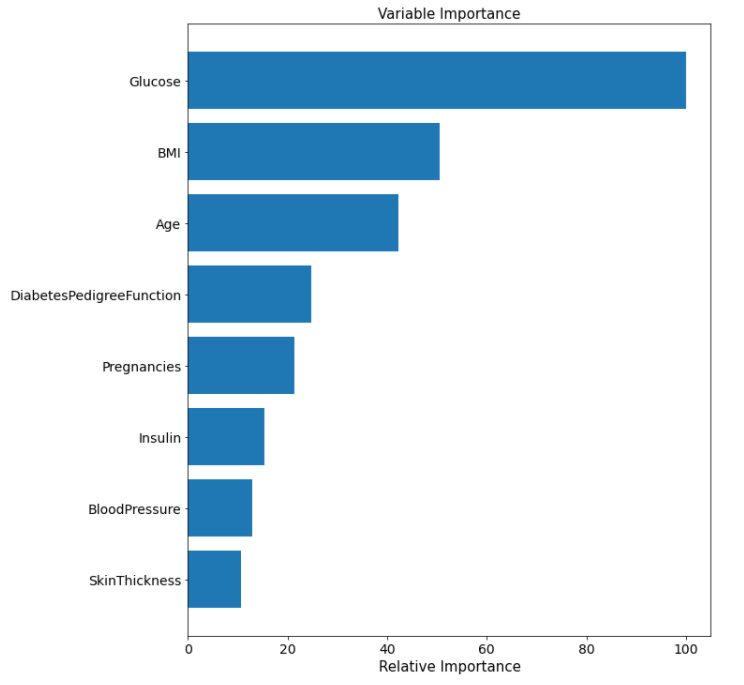## Possible Interview Questions

Random Forest is one of the coolest and favorite algorithms in Machine Learning. A more comprehensive range of applications can be seen built using this algorithm, and hence considered to be one of the important topics to be asked in machine learning interviews,

1. Explain the working of Random Forest.
2. What is an out-of-bag error, and why is it considered one of the better choices to test the random forest model?
3. What are the disadvantages of Random Forest?
4. What is the difference between Bagging and Random Forest?
5. Explain the Bootstrapping process.
6. What is an ensemble process, and why is Random Forest an ensemble approach?

## Conclusion

This tutorial taught us the basic understanding of the decision tree and its working. We also learned the working of Random Forest and its advantages over decision trees. Finally, we learned model building, evaluation, hyper-parameter tuning, and finding essential features using Sk-learn. We hope you enjoyed the article.

Enjoy learning, Enjoy algorithms!